# Regular Totally Separable Sphere Packings arXiv .pdf

### File information

This PDF 1.5 document has been generated by LaTeX with hyperref package / pdfTeX-1.40.12, and has been sent on pdf-archive.com on 06/06/2015 at 20:21, from IP address 70.72.x.x. The current document download page has been viewed 611 times.
File size: 433.98 KB (10 pages).
Privacy: public file

### Document preview

Regular Totally Separable Sphere Packings

arXiv:submit/1273446 [math.MG] 6 Jun 2015

Samuel Reid∗
June 6, 2015

Abstract
The topic of totally separable sphere packings is surveyed with a focus on regular
constructions, uniform tilings, and contact number problems. An enumeration of all
regular totally separable sphere packings in R2 , R3 , and R4 which are based on convex
uniform tessellations, honeycombs, and tetracombs, respectively, is presented, as well
as a construction of a family of regular totally separable sphere packings in Rd that is
not based on a convex uniform d-honeycomb for d ≥ 3.

Keywords: sphere packings, hyperplane arrangements, contact numbers, separability.
MSC 2010 Subject Classifications: Primary 52B20, Secondary 14H52.

1

Introduction

In the 1940s, P. Erd¨os introduced the notion of a separable set of domains in the plane,
which gained the attention of H. Hadwiger in . G.F. T´oth and L.F. T´oth extended this
notion to totally separable domains and proved the densest totally separable arrangement
of congruent copies of a domain is given by a lattice packing of the domains generated by
the side-vectors of a parallelogram of least area containing a domain . Totally separable
domains are also mentioned by G. Kert´esz in , where it is proved that a cube of volume V
contains a totally separable set of N balls of radius r with V ≥ 8N r3 . Further results and
references regarding separability can be found in a manuscript of J. Pach and G. Tardos .
This manuscript continues the study of separability in the context of regular unit sphere
packings, i.e., infinite sets of unit spheres
P=

[

xi + Sd−1



i=1

University of Calgary, Centre for Computational and Discrete Geometry (Department of Mathematics &amp; Statistics), and Thangadurai Group (Department of Chemistry), Calgary, AB, Canada.
e − mail : smrei@ucalgary.ca.

1

in Rd with kxi − xj k ≥ 2, whose contact graphs GP = (V, E), where V = {xi | i ∈ N} and




E = (i, j) | xi + Sd−1 ∩ xj + Sd−1 6= ∅ ,
are regular (every vertex has equal degree); this means that every sphere in the packing
touches the same number of spheres.
Let C(Pn ) be the contact number of a unit sphere packing Pn with n spheres, i.e., the
cardinality of the edge set of the contact graph GPn . Determining the maximum contact
number of a unit sphere packing with n spheres is known as the contact number problem.
The contact number problem√for circle packings in R2 was solved exactly in 1974 by H.
Harborth in  to be b3n − 12n − 3c. Upper and lower bounds on the contact number
problem for finite packing of unit balls in R3 were provided by K. Bezdek and the author
in  and studied in detail up to n = 18 by M. Holmes-Cerfon in  improving the lower
bounds for some values. Consult  and references therein for more information regarding
contact numbers of unit sphere packings and arrangements of spheres in higher dimensions.
Definition 1. A sphere packing P is totally separable if every tangent hyperplane to a pair
of touching spheres has an empty intersection with the interior of all spheres in P.
The contact number problem for totally separable sphere packings is studied and all regular totally separable sphere packings in R2 , R3 , and R4 based on convex uniform tessellations
(classified in an unpublished manuscript of G. Olshevsky ) are constructed. Now, let
c(n, d) =

max C(Pn ),

sep(Pn )=1

where sep(·) is a measure on sphere packings called the separability of the packing which is
defined formally in the appendix; intuitively, the separability of a packing is 0 if the packing
is inseparable and 1 if it is totally separable. The theory of minimal area polyominoes
developed in  is used with Euler’s formula to provide a proof of the contact number
problem for totally separable circle packings:
j
√ k
c(n, 2) = 2(n − n) .
Furthermore, heuristics are provided for the upper bound on the contact number problem for
totally separable sphere packings in Rd which is based
√on the number of edges of polyominoes
over the cubic d-honeycomb and hence exact when d n ∈ N:
j 
k
d−1
c(n, d) ≤ d n − n d
.
As this manuscript was being prepared, K. Bezdek, B. Szalkai, and I. Szalkai proved the
above upper bound on c(n, d) with an ingenious argument involving box-polytopes and the
isoperimetric inequality . The paper ends with a construction of a family of regular
totally separable sphere packings in Rd that is not based on a convex uniform tessellation
for d ≥ 3 and an outline of future research directions.
The most basic example of when the condition on a totally separable sphere packing is
violated is explained in the form of a lemma for future reference.
2

Lemma 1. If the contact graph GP of a sphere packing P in Rd contains a k-simplex for
2 ≤ k ≤ d, then P is not totally separable.
Proof. First consider the case where GP contains a 2-simplex and observe that it violates
total separability. For, the tangent line generated by the touching circles associated with an
edge e of the 2-simplex intersect the interior of the circle associated with the vertex which
is not an endpoint of e. Proceed by induction, observing from the base case d = 2 that
any k-simplex with 3 ≤ k ≤ d in GP violates total separability as that k-simplex contains a
2-simplex somewhere in its flag, thus proving the lemma.
This lemma will be used extensively for classifying totally separable sphere packings
based on convex uniform tesselations of Rd , also known as tilings or honeycombs.

2

Regular Totally Separable Circle Packings in R2

Regular totally separable circle packings in R2 which are based on convex uniform tilings
are classified by the following theorem.
Theorem 1. There are exactly 4 convex uniform tilings in R2 which generate totally separable circle packings:
1. P1 - Square tiling, {4, 4, 4}
2. P3 - Hexagonal tiling, {6, 6, 6}
3. K6 - Truncated square tiling, {4, 8, 8}
4. K9 - Omnitruncated trihexagonal tiling, {4, 6, 12}
Proof. Apply Lemma 1 to the list of 11 convex uniform tilings of R2 ; three Pythagorean
tilings and eight Keplerian tilings . Clearly, if P is a 4-regular totally separable packing
of unit circles in R2 generated by a convex uniform tiling, then P is congruent to P1. If P is a
3-regular totally separable packing of unit circles in R2 generated by a convex uniform tiling,
then P is congruent to P3, K6, K9 or a subset of P1. If P is a 2-regular totally separable
packing of unit circles in R2 generated by a convex uniform tiling, then P is congruent to a
subset of either P1, P3, K6, or K9.
The theory of minimal area polyominoes and Euler’s formula is used to provide an exact
solution to the contact number problem for totally separable circle packings; an alternative
explicit proof, not relying on the results of , which extends a proof technique of H. Harborth
 appears in .
Theorem 2. Given n ∈ N, there exists a totally separable circle packing Pn in R2 with
contact number
j
√ k
C(Pn ) = 2(n − n) .
Furthermore, no totally separable circle packing in R2 has a larger contact number.
3

Proof. By Euler’s formula, n − (|E| + P (c)) + a = 2, where |E| is the cardinality of the
edge set of the contact graph GPn , P (c) is the perimeter of the polyomino c with area a
generated by placing n unit 2-cubes so that elements of Pn are incircles. Interpolate the
piece-wise defined function from Corollary 2.5 of  which provides the minimal perimeter
of a polyomino of area a in order to obtain the desired formula.

Figure 1: A finite part of the contact graph, convex uniform tiling, and 3-regular totally
separable circle packing generated by the truncated square tiling.

4

3

Regular Totally Separable Sphere Packings in R3

Regular totally separable sphere packings in R3 which are based on convex uniform honeycombs are classified by the following theorem.
Theorem 3. There are exactly 7 convex uniform honeycombs in R3 which generate totally
separable sphere packings in R3 :
1. J 1 - Cubic honeycomb
2. J 3 - Hexagonal prismatic honeycomb
3. J 6 - Truncated square prismatic honeycomb
4. J 9 - Omnitruncated trihexagonal prismatic honeycomb
5. J 16 - Bitruncated cubic honeycomb
6. J 18 - Cantitruncated cubic honeycomb
7. J 20 - Omnitruncated cubic honeycomb
Proof. Apply Lemma 1 to N. Johnson’s list of 28 convex uniform honeycombs . Clearly,
if P is a 6-regular totally separable packing of unit spheres in R3 generated by a convex
uniform honeycomb, then P is congruent to J 1. If P is a 5-regular totally separable packing
of unit spheres in R3 generated by a convex uniform honeycomb, then P is congruent to J 3,
J 6, J 9, or a subset of J 1. If P is a 4-regular totally separable packing of unit spheres in
R3 generated by a convex uniform honeycomb, then P is congruent to J 16, J 18, J 20, or a
subset of either J 1, J 3, J 6, or J 9. If P is a 3-regular, or 2-regular totally separable packing
of unit spheres in R3 generated by a convex uniform honeycomb, then P is congruent to a
subset of either J 1, J 3, J 6, J 9, J 16, J 18, or J 20.

4

Regular Totally Separable Sphere Packings in R4

Regular totally separable sphere packings in R4 based on convex uniform 4-honeycombs are
classified by the following theorem.
Theorem 4. There are exactly 18 convex uniform tetracombs in R4 which generate totally
separable sphere packings in R4 :
1. O1 - Tesseractic tetracomb
2. O3 - Square-hexagonal duoprismatic tetracomb
3. O6 - Tomosquare-square duoprismatic tetracomb
4. O9 - Omnitruncated-trihexagonal-square duoprismatic tetracomb
5

5. O16 - Bitruncated-cubic prismatic tetracomb
6. O18 - Cantitruncated-cubic prismatic tetracomb
7. O20 - Omnitruncated-cubic prismatic tetracomb
8. O39 - Hexagonal duoprismatic tetracomb
9. O42 - Hexagonal-tomosquare duoprismatic tetracomb
10. O45 - Hexagonal-omnitruncated-trihexagonal duoprismatic tetracomb
11. O63 - Tomosquare duoprismatic tetracomb
12. O66 - Tomosquare-omnitruncated-trihexagonal duoprismatic tetracomb
13. O78 - Omnitruncated-trihexagonal duoprismatic tetracomb
14. O99 - Truncated icositetrachoric tetracomb
15. O100 - Great diprismatotesseractic tetracomb
16. O103 - Omnitruncated tesseractic tetracomb
17. O132 - Omnitruncated icositetrachoric tetracomb
18. O140 - Great-prismatodecachoric tetracomb
Proof. Apply Lemma 1 to G. Olshevsky’s list of 143 convex uniform 4-honeycombs .
Clearly, if P is a 8-regular totally separable packing of unit spheres in R4 generated by a
convex uniform tetracomb, then P is congruent to O1. If P is a 7-regular totally separable
packing of unit spheres in R4 generated by a convex uniform tetracomb, then P is congruent
to O3, O6, O9, or a subset of O1. If P is a 6-regular totally separable packing of unit
spheres in R4 generated by a convex uniform tetracomb, then P is congruent to O16, O18,
O20, O39, O42, O45, O63, O66, O78, or a subset of either O1, O3, O6, or O9. If P is
a 5-regular totally separable packing of unit spheres in R4 generated by a convex uniform
tetracomb, then P is congruent to O99, O100, O103, O132, O140, or a subset of either
O1, O3, O6, O9, O16, O18, O20, O39, O42, O45, O63, O66, or O78. If P is a 4-regular,
3-regular, or 2-regular totally separable packing of unit spheres in R4 generated by a convex
uniform tetracomb, then P is congruent to a subset of either O1, O3, O6, O9, O16, O18,
O20, O39, O42, O45, O63, O66, O78, O99, O100, O103, O132, or O140.
The regularity of each 4-honeycomb is determined by inspecting the number of vertices
of the vertex figure associated with the honeycomb, e.g., the vertex figure of O100 is an
irregular pentachoron, implying that the 4-dimensional sphere packing generated by the
great diprismatotessseractic tetracomb is 5-regular.

6

5

Totally Separable Sphere Packings in Rd

Totally separable sphere packings in Rd are studied and future research directions are outlined. The following heuristics for the upper bound to the contact number problem for totally
separable sphere packings in Rd provide a reasonable intuitive explanation of the following
theorem.
From the formula for the number of m-cubes on the boundary of a d-cube for m = 1
observe that
  j 
k j 
k
d−1
d−1
d−1 d
= d n−n d
= d 2d − (2d ) d
2
1

for n = 2d . Similarly, for any n = d k ∈ N there is a k
| × k ×{z· · · × k} d-cube with
d times
j 
k
d
d d−1
d
d k − (k )
edges, implying that the upper bound in the following theorem is an
equality. Assume that k d &lt; n &lt; (k + 1)d and observe that the upper bound on c(n, d)
overestimates the supremum over edge cardinalities of (k + δ1 ) × (k + δ2 ) × · · · × (k + δd )
unit polyominoes with n cells, where δi ∈ {0, 1}.
Theorem 5. For n ∈ N,
with equality when

d

k
j 
d−1
,
c(n, d) ≤ d n − n d

n ∈ N.

Proof. Improving upon an earlier and lengthier unpublished case analytic proof, K. Bezdek,
B. Szalkai, and I. Szalkai provide an elegant proof using box-polytopes and the isoperimetric
inequality .
The classification of uniform d-honeycombs is incomplete, leading to great difficulty in
establishing the above characterizations of totally separable sphere packings in d = 2, 3, 4 for
d ≥ 5. The ongoing work by J. Bowers, G. Olshevsky, N. Johnson, and others of classifying
uniform polyterons will soon result in the complete classification of uniform 5-honeycombs,
and the study of uniform polypetons generating uniform 6-honeycombs has only recently
begun. For d ≥ 7 there appears to be no significant work on uniform honeycombs; although
R. Klitzing has classified certain uniform polytopes up to d = 8 . Future research
on the topic of regular totally separable sphere packings should include a comprehensive
construction of families of k-regular totally separable sphere packings in Rd for 3 ≤ k ≤ 2d−1
and d ≥ 5. These are the unknown bounds on k-regularity because for k = 2 spheres can be
placed along an apeirogon (infinite line with evenly spaced points) and for k = 2d spheres
can be placed on the cubic d-honeycomb. For an example to motivate future research in this
direction, a construction in Rd of a (d + 1)-regular totally separable sphere packing which is
not based on a convex uniform d-honeycomb for d ≥ 3 is presented. A similar construction
would be desired for 3 ≤ k ≤ d and d + 2 ≤ k ≤ 2d − 1; regardless of whether or not it is
based on a convex uniform d-honeycomb.

7

Theorem 6. There exists a (d + 1)-regular totally separable sphere packing in Rd for d ≥ 3
which is not based on a convex uniform d-honeycomb.


Proof. Let Qd0 = conv x0,1 , ..., x0,2d be a unit d-cube in Rd and place 2d unit d-cubes


Qd1 = conv x1,1 , ..., x1,2d
..
.


Qd2d = conv x2d ,1 , ..., x2d ,2d
so that kx0,1 − x1,1 k = 1, ..., kx0,2d − x2d ,1 k = 1 with xi,1 lying outside Qd0 along a line
emanating from the centroid of Qd0 through x0,i for 1 ≤ i ≤ 2d . Now construct
P2d +4d =

2d[
+4d [
2d

xi,j + Sd−1



i=1 j=1

and iteratively place 2d −1 unit d-cubes diagonally out of each existing unit d-cube Qd1 , ..., Qd2d
as above so that spheres may be placed around their vertices which generate a packing congruent to P2d +4d . Indefinitely extending this procedure leads to an infinite totally separable
sphere packing which is (d + 1)-regular. For, let x + Sd−1 be an arbitrary sphere in this
packing and observe that it touches d other spheres placed on adjacent vertices of the unit
d-cube which x is a vertex of, and also touches 1 other sphere which is diagonally outward
as in the construction. Furthermore, for d = 2 this corresponds to the truncated square
tiling K6 and for d ≥ 3 this corresponds to a scaliform which contains an elongated cubic
bifrustum.
The classification of regular totally separable sphere packings which are not based on
convex uniform 3-honeycombs is then a sub-problem of classifying all scaliforms (vertextransitive honeycombs) in R3 ; from a simplex-free scaliform in R3 one can construct a totally
separable sphere packing by placing equal size spheres at the vertices. The questionable
existence of aperiodic totally separable sphere packings in any dimension remains unexplored.
Conjecture 1. No aperiodic totally separable sphere packing exists in any dimension.

Appendix: Separability as a Geometric Measure
Separability is introduced as a geometric measure where inseparable sphere packings have
a separability of 0 and totally separable sphere packings have a separability of 1. Let He
denote the tangent hyperplane to a pair of touching spheres in Rd associated with edge e
of the contact graph GP = (V, E). First define the separability measure for finite sphere
packings Pn with GPn = (Vn , En ) by



X He | He ∩ int xi + Sd−1 = ∅, 1 ≤ i ≤ n
.
sep(Pn ) =
|E
n|
e∈E
n

8

If a sphere packing P ,→ Rd can be constructed so that P = limn→∞ Pn for some sequence
of finite sphere packings Pn , then



X He | He ∩ int xi + Sd−1 = ∅, 1 ≤ i ≤ n
.
sep(P) = lim
n→∞
|En |
e∈E
n

Observe that if every tangent hyperplane He at a contact point associated with the edge e
intersects the interior of another sphere in the packing P then sep(P) = 0 and similarly if
none intersect the interior of a sphere in the packing then sep(P) = 1; in the former case P
is called inseparable and in the latter case P is called totally separable.

6

Acknowledgements

Many thanks are to my first supervisor K´aroly Bezdek for introducing me to the topic
of totally separable sphere packings and having so many discussions with me regarding
geometry research over the years. Special thanks are also to Jonathan Bowers for helping to
check through George Olshevsky’s 143 honeycombs for realizable packings; three of which
remained unnoticed to me in the compilation of Theorem 4.

References
 H. Hadwiger. Nonseparable convex systems. Amer. Math. Monthly: 1947, vol: 54, pp:
583 - 585.
 G.F. T´oth. On totally separable domains. Acta mathematica Hungarica: 1973, vol: 24,
pp: 229 - 232.
 G. Kert´esz. On totally separable packings of equal balls. Acta mathematica Hungarica:
1988, vol: 51, pp: 363 - 364.
 J. Pach and G. Tardos. Separating Convex Sets By Straight Lines. Discrete Mathematics: 2001, vol: 24, pp: 427 - 433.
 H. Harborth. L¨osung zu Problem 664A. Elem. Math. 29 (1974), 14-15.
 K. Bezdek and S. Reid. Contact graphs of unit sphere packings revisited Journal of
Geometry: April 2013, vol: 104, pp: 57 - 83.
 M. Holmes-Cerfon. Enumerating nonlinearly rigid sphere packings. arXiv:1407.3285
(2014).
 K. Bezdek. Lectures on Sphere Arrangements - the Discrete Geometric Side. Springer,
2013.

9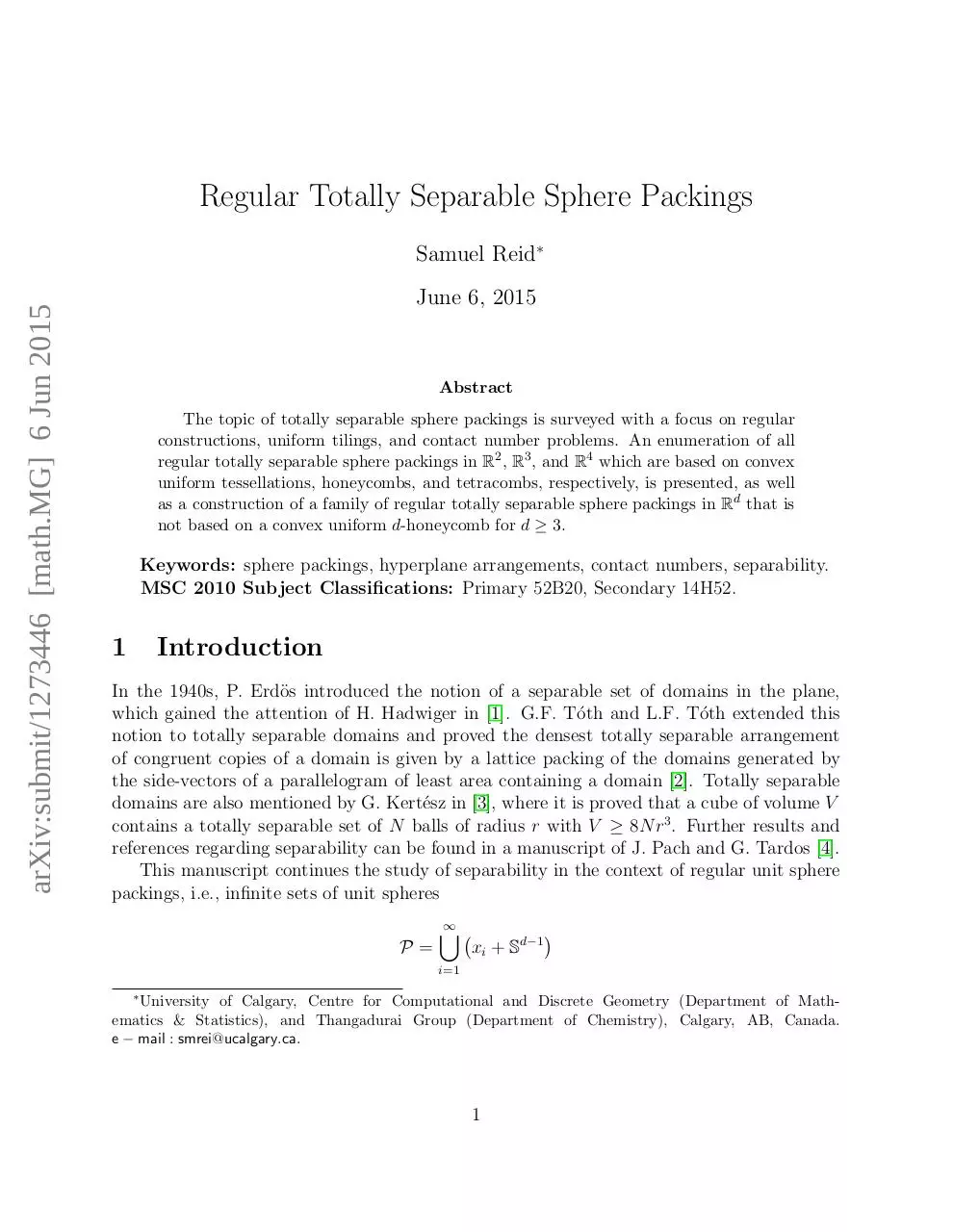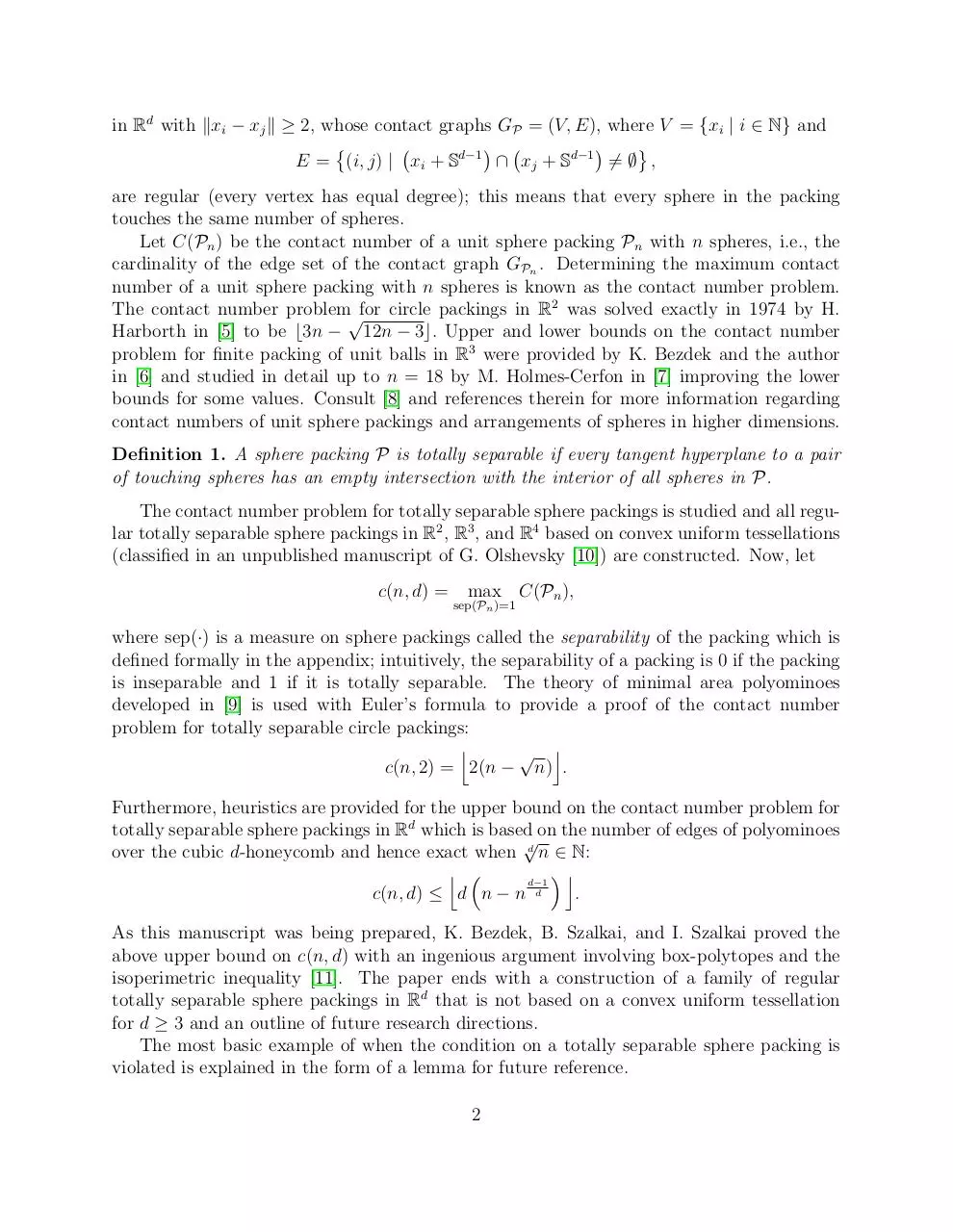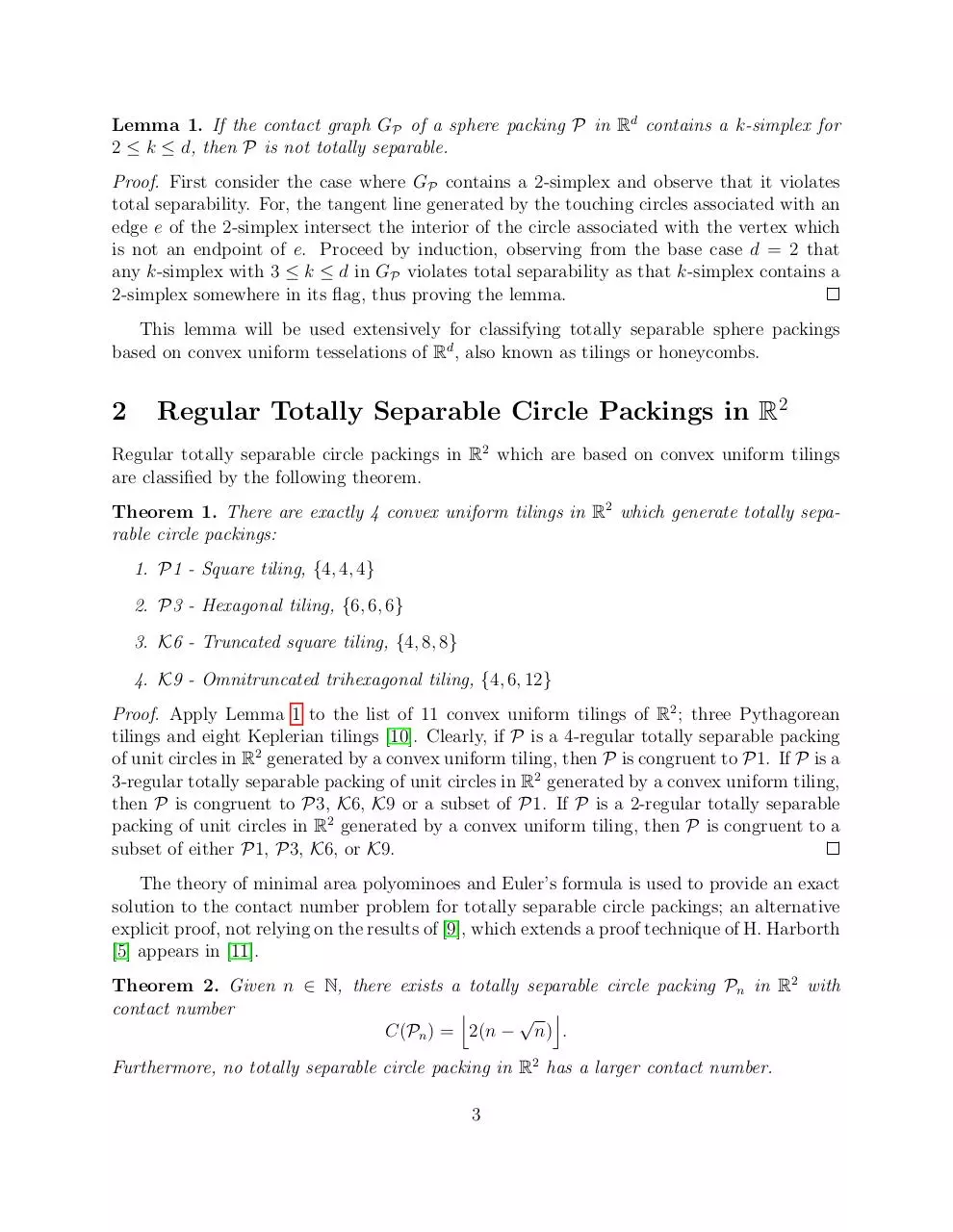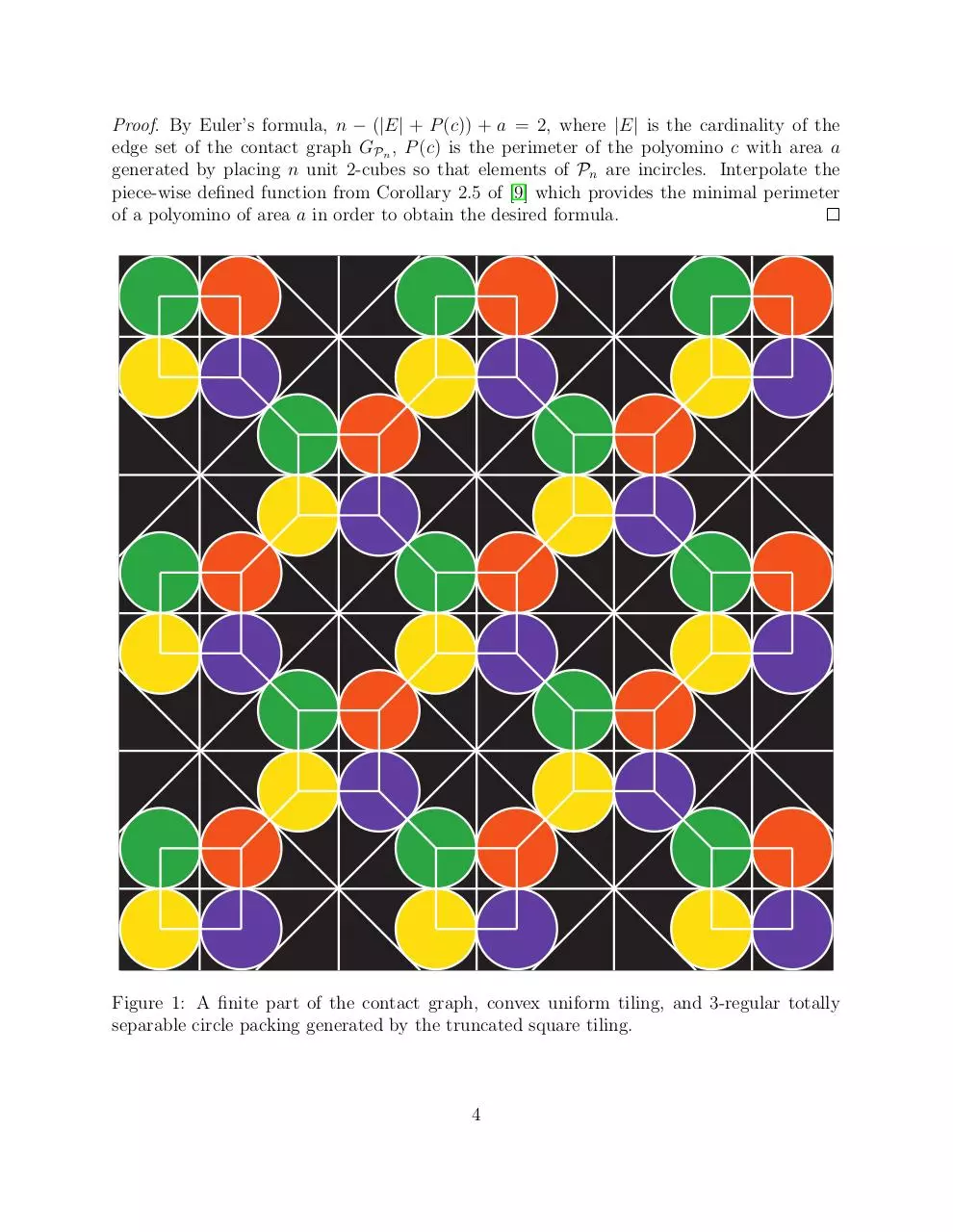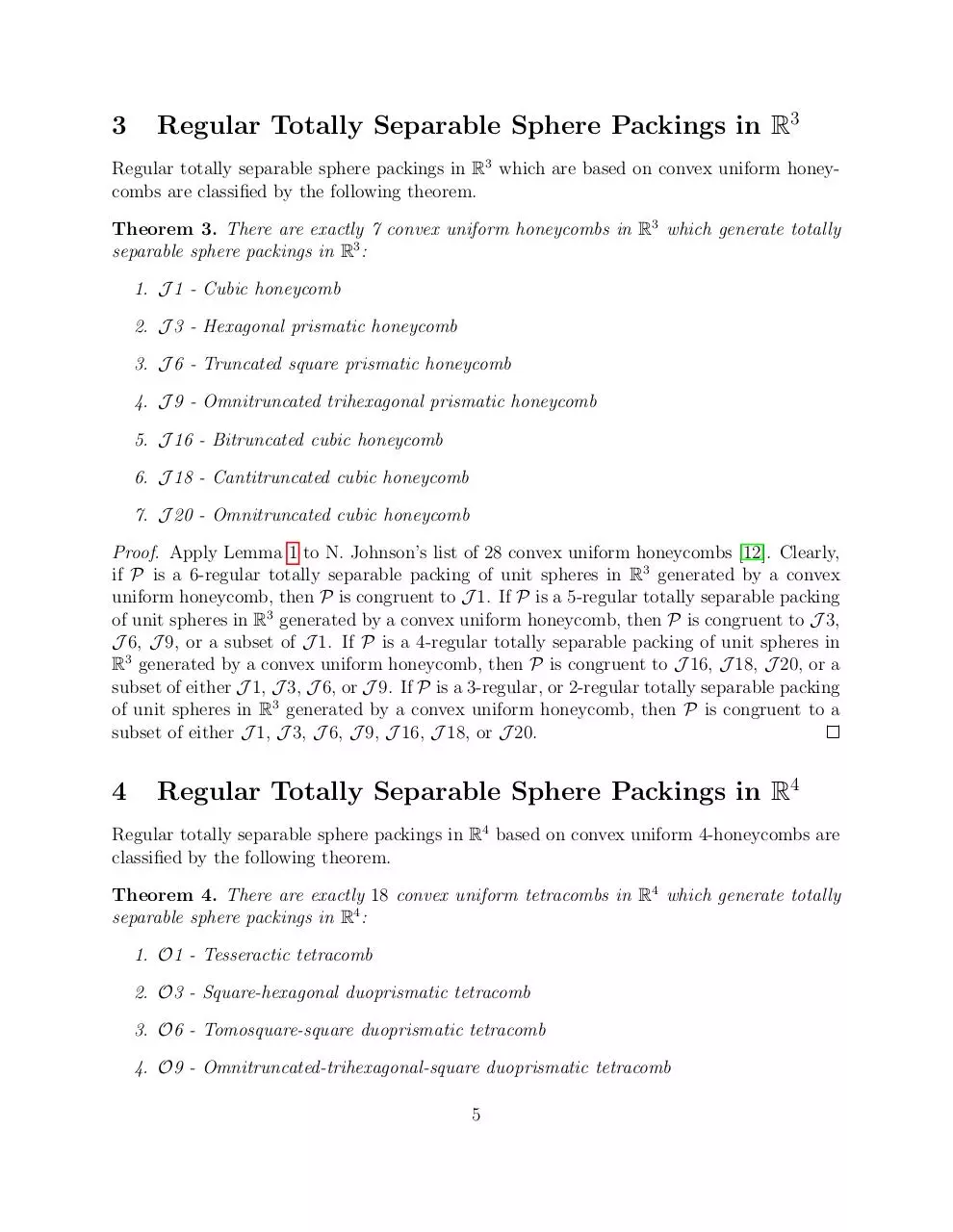Regular Totally Separable Sphere Packings arXiv.pdf (PDF, 433.98 KB)

### Share on social networks

#### HTML Code

Copy the following HTML code to share your document on a Website or Blog